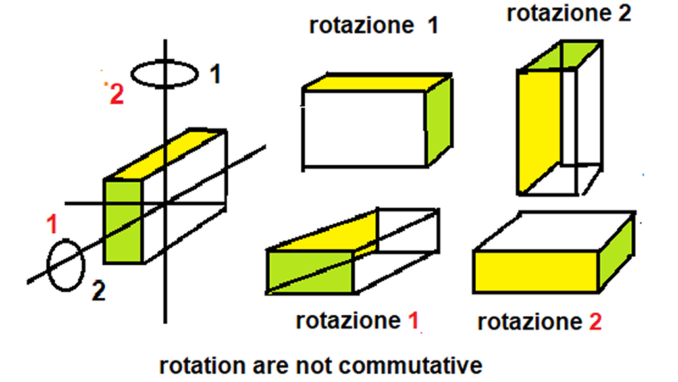# CommutativitySommacal alfonso [CC BY-SA 4.0], via Wikimedia CommonsA binary operation is said to commute if the order of the operands does not alter the result.

In the real numbers, $$\Bbb R$$, the following commutative laws hold :

$$x + y = y + x,$$ for all $$x,y \in \Bbb R$$

$$xy = yx,$$ for all $$x,y \in \Bbb R$$

Although these laws seem elemental, we wish to emphasise the fundamental nature of this property in the arithmetic of the real numbers.

The operation of addition is commutative.

$$3 + 5 = 5 + 3 = 8$$

The order in which we add does not alter the result.

The operation of subtraction is non commutative.

$$3-5 = -2\\ 5-3 = 2$$

When we change the order of the operands we obtain different solutions.

Multiplication is commutative.

$$2 \times 10 = 10 \times 2 = 20$$

Division is non commutative.

$$2 \div 10 = 1/5\\ 10 \div 2 = 5$$

We have other entities that have real numbers as elements where the commutative multiplication law does not hold.

Multiplication of square matrices $$(n \times n)$$ is non commutative.

$$\mathbf A \mathbf B \neq \mathbf B \mathbf A$$

Note that upper-case, boldface, upright letters denote matrices.

Scalar multiplication of two vectors (the dot product) is commutative.

$$\mathbf a \cdot \mathbf b = \mathbf b \cdot \mathbf a$$

Vector multiplication of two vectors (the cross product) is non commutative.

$$\mathbf a \times \mathbf b \neq \mathbf b \times \mathbf a$$

Lower-case, boldface, upright letters denote vectors.

Commutativity holds for addition and multiplication when we extend the number system from the real numbers, $$\Bbb R$$, to the complex numbers, $$\Bbb C$$.

The complex numbers allow us to rotate in two dimensions.

In order to rotate in three dimensions the number system is extended to the quaternion numbers, $$\Bbb H$$, where the commutative law of multiplication does not hold.

Order matters.

© OldTrout $$2019$$